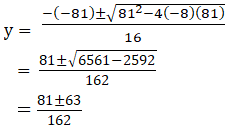Find the sum of the terms of an infinite decreasing G.P. in which all the terms are positive, the first term is 4, and the difference between the third and fifth term is equal to $$\dfrac{32}{81}$$.

Asked by Aaryan | 1 year ago |  33

##### Solution :-

Let ‘a’ be the first term of GP and ‘r’ be the common ratio.

We know that nth term of a GP is given by-

an = arn-1

As, a = 4 (given)

And a5 – a3 = $$\dfrac{32}{81}$$ (given)

4r4 – 4r2 = $$\dfrac{32}{81}$$

4r2(r2 – 1) = $$\dfrac{32}{81}$$

r2(r2 – 1) = $$\dfrac{8}{81}$$

Let us denote r2 with y

81y(y-1) = 8

81y2 – 81y – 8 = 0

Using the formula of the quadratic equation to solve the equation, we gety = $$\dfrac{18}{162}= \dfrac{1}{9}$$ or

y = $$\dfrac{8}{9}$$

So, r2 = $$\dfrac{1}{9}$$ or $$\dfrac{8}{9}$$

$$\dfrac{1}{3}$$

We know that,

Sum of infinite, S = $$\dfrac{ a}{(1 – r)}$$

Where, a = 4, r = $$\dfrac{1}{3}$$

$$\dfrac{12}{2}$$

= 6

Sum of infinite, S =$$\dfrac{ a}{(1 – r)}$$

Where, a = 4, r = $$\dfrac{2}{\sqrt{\dfrac{2}{3}}}$$

$$S_∞ = \dfrac{4}{\dfrac{2}{2\sqrt{3}}}$$

= $$\dfrac{12}{3-2\sqrt{2}}$$

Answered by Aaryan | 1 year ago

### Related Questions

#### Construct a quadratic in x such that A.M. of its roots is A and G.M. is G.

Construct a quadratic in x such that A.M. of its roots is A and G.M. is G.

#### Find the two numbers whose A.M. is 25 and GM is 20.

Find the two numbers whose A.M. is 25 and GM is 20.

#### If a is the G.M. of 2 and 1/4 find a.

If a is the G.M. of 2 and $$\dfrac{1}{4}$$ find a.

#### Find the geometric means of the following pairs of numbers

Find the geometric means of the following pairs of numbers:

(i) 2 and 8

(ii) a3b and ab3

(iii) –8 and –2

Insert 5 geometric means between $$\dfrac{32}{9}$$ and $$\dfrac{81}{2}$$.